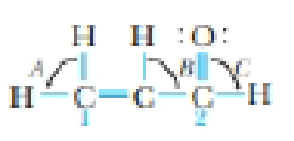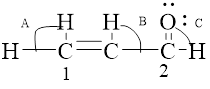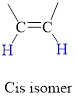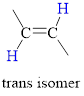Chapter 9, Problem 36GQ

Chapter
Section
Textbook Problem

Acrolein, a component of photochemical smog, has a pungent odor and irritates eyes and mucous membranes.(a) What are the hybridizations of carbon atoms 1 and 2?(b) What are the approximate values of angle A, B, and C?(c) Is cis-trans isomerism possible here?

(a)

Interpretation Introduction

Interpretation:

Hybridizations of carbon atoms 1 and 2 in acrolein has to be determined.Concept Introduction:

Hybridization is the mixing of valence atomic orbitals to get equivalent hybridized orbitals that having similar characteristics and energy.

Geometry of a molecule can be predicted by knowing its hybridization.

sp3  hybrid orbitals is produced by hybridization of single s-orbital and three p-orbital.

sp2  hybrid orbitals is produced by hybridization between one s-orbital and two p-orbitals; molecules with double bond possess this type of hybridization.

sp  hybrid orbitals is produced by hybridization of single s-orbital and single p-orbital.

Explanation

Hybridizations of carbon atoms 1 and 2 in acrolein is determined and indicated below,

(b)

Interpretation Introduction

Interpretation:

Approximate values of angles A,B and C in the  given acrolein molecule has to be determined.Concept Introduction:

Hybridization is the mixing of valence atomic orbitals to get equivalent hybridized orbitals that having similar characteristics and energy.

Geometry of a molecule can be predicted by knowing its hybridization.

sp3  hybrid orbitals is produced by hybridization of single s-orbital and three p-orbital.

sp2  hybrid orbitals is produced by hybridization between one s-orbital and two p-orbitals; molecules with double bond possess this type of hybridization.

sp  hybrid orbitals is produced by hybridization of single s-orbital and single p-orbital.

TypeofmoleculeHybridaizationGeometryBondangleAX2spLinear180°AX3,AX2Bsp2Trigonalplanar120°AX4,AX3B,AX2B2sp3Tetrahedral109.5°AX5,AX4B,AX3B2,AX2B3sp3dTrigonalbipyramidal120°,90°AX6,AX5B,AX4B2sp3d2Octahedral90°ACentralatomXAtomsbondedtoABNonbondingelectronpairsonA

(c)

Interpretation Introduction

Interpretation:

It should be checked that whether the given molecule can form cis-trans isomers or not.Concept Introduction:

Isomer: The molecule has same molecular formula and attached for different functional groups it is called isomers.

Cis Isomer: The two groups attached on same side of C=C double bond irrespective of molecules or atoms is called cis isomer this type molecule has polar nature.

In cis isomer the hydrogen atoms are on the same side of the two carbon atom.Trans isomer: The two groups are attached on opposite sides of the carbon-carbon double bond;.

The hydrogen atoms are on the opposite side of the two carbon atom.Still sussing out bartleby?

Check out a sample textbook solution.

See a sample solution

The Solution to Your Study Problems

Bartleby provides explanations to thousands of textbook problems written by our experts, many with advanced degrees!

Get Started

Find more solutions based on key concepts